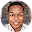# BECE 2010 Mathematics Paper 1OBJECTIVE TEST

Question 1: Which of the following sets is well defined?
A. {Man, Kofi, Red, 14}
B. {Ink, Mango, Green, Nail}
D. {Seth, Mary, Jacob, Evelyn}

Question 2: If set B is a subset of set A, then
A. sets A and B have the same number of elements
B. some members of set B can be found in set A
C. no member of set B is in set A
D. all the members of set B are in set A

Question 3: The least common multiple (LCM) of 16, 30 and 36 is
A. 3
B. 6
C. 240
D. 720

Question 4:

Question 5: The numbers 32, 33, 34,.........,.........., 42 form a sequence in base 5.
Find the missing numbers
A. 35, 36
B. 30, 31
C. 40, 41
D. 31, 41

Question 6:
Question 7: Find the total cost of 25 pens and 75 books if each pen costs GH¢ 0.20 and each book costs GH¢ 0.30.
A. GH¢22.50
B. GH¢23.50
C. GH¢27.50
D. GH¢50.00

Question 8: Simplify     - 27 + 18 - (10 - 14) - (-2)
A. -3
B. -7
C. -11
D. -35

Question 9: Arrange the following numbers from the lowest to the highest: 0.5, 3, -5, 0.
A. 0, 0.5, -5, 3
B. 0, -5, 0.5, 3
C. -5, 0, 0.5, 3
D. -5, 0.5, 0, 3

Question 10:
Question 11: Find the value of 124.3 + 0.275 + 74.06, correcting your answer to one decimal place.
A. 198.6
B. 198.7
C. 892.0
D. 892.4

Question 12: Esi and Kwasi are 12 and 8 years old respectively. They share 60 mangoes in the ratio of their ages. How many mangoes does Esi get?
A. 42
B. 40
C. 36
D. 18

Question 13: It takes 6 students 1 hour to sweep their school compound. How long will it take 15 students to sweep the same compound?
A. 24 minutes
B. 12 minutes
C. 3 hours
D. 2 hours

Question 14: A housing agent makes a commission of GH¢ 103,500 when he sells a house for GH¢ 690,000. Calculate the percentage of his commission.
A. 15.0%
B. 10.0%
C. 7.5%
D. 5.0%

Question 15: A simple interest of GH¢ 37,500.00 is earned on an amount of GH¢ 500,000.00 for 3 years. Find the rate of interest per annum.
A. 20.0%
B. 10.0%
C. 5.0%
D. 2.5%

Question 16: Question 17: The scores of 10 students in an examination are given as follows: 45, 12, 75, 81, 54, 51, 24, 67, 19 and 39.
What is the median of the scores?
A. 39
B. 48
C. 51
D. 54

Question 18: Question 19: Eighteen cards are numbered from 11 to 29. If one card is chosen at random, what is the probability that it contains the digit 2?
A. ³∕₉
B. ⁷∕₁₈
C. ⁵∕₉
D. ¹¹∕₁₉

Question 20: Question 21: Factorize: xy + 5x + 2y + 10.
A. (x + 5)(2y + 10)
B. (x + 2)(y + 10)
C. (x + 5)(y + 2)
D. (x + 2)(y + 5)

Question 22: If x = {2, 3, 4, 5}, find the truth set of 2x + 1 < 8.
A. {2, 3, 4}
B. {2, 3}
C. {3, 4}
D. {4, 5}

Question 23: Solve the inequality: 7x -(10x+ 3) ≥ -9
A. x≥2
B. x≤4
C. x≥4
D. x≤2

Question 24: Question 25: Find the circumference of a circle whose area is equal to 64π cm²·
A. 32π cm
B. 16π cm
C. 8π cm
D. 4π cm

Question 26: Which of the following geometric figures is the plane shape of a cube?
A. Circle
B. Rectangle
C. Square
D.  Triangle

Question 27: How many lines of symmetry has a rectangle?
A. 4
B. 3
C. 2
D. 1

Question 28: A rectangular box has length 20 cm, width 6cm and height 4 cm. Find how many cubes of size 2 cm that will fit into the box.
A. 120
B. 60
C. 30
D. 15

Question 29: The interior angle of a regular polygon is 120°. How many sides has this polygon?
A. 3
B. 4
C. 5
D. 6

Question 30: Question 31: Question 32: Express 7 min. 30 sec. as a percentage of 1 hour.
A. 2.5%
B. 7.5%
C. 11.7%
D. 12.5%

Question 33: Question 34:
Question 35: Question 36: Express 4037 in standard form.
A. 4.037 x 10-⁴
B. 4.037 x 10-³
C. 4.037 x 10³
D. 4.037 x 10⁴

Question 37:
Question 38: Given that vector a = (-5 12) and b = (10x 12) find the value of x if a = b.
A. -2
B. -½
C. ½
D. 2

Question 39:
Question 40: Ama is 9 years older than Kwame. If Kwame is 18 years old, find the ratio of the age of Kwame to that of Ama.
A. 3 : 2
B. 1 : 3
C. 2 : 3
D. 2 : 1